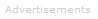# Definition of phase rule

One area often encountered in thermodynamics is the description of the equilibrium state of a system. We can describe a system as being in a reproducible equilibrium state when we can fix as constant some number of intensive variables. As a simple example, if an evacuated container is filled to half its volume with pure water, we would expect the system to reach equilibrium when the temperature, T, becomes constant and uniform throughout the container. Our common sense tells us that only this one intensive variable must be uniform throughout the system for the system to be at equilibrium. The Phase Rule

At equilibrium the number of independent variables (also called thermodynamic potentials) that must be fixed is called the number of degrees of freedom, F. It is given by:

F = C - p + 2

where C is the number of components and p is the number of phases in the system. For our simple example above, there is one component and two phases, liquid water and water vapor, so the number of degrees of freedom is:

F = 1 - 2 + 2 = 1

As expected above, only one variable must be constant, T in the example, to determine that the system is at equilibrium.

The number of components can sometimes be tricky to determine. if there is a fixed relationship between chemical species, they are not separate components. For example, if sodium chloride is added to water you would not count Na+ and Cl- as separate components. Since [Na+] equals [Cl-], there is only one additional component, salt.

if we repeat the above example of partially filling the evacuated container using salt water, we now have:

F = 2 - 2 + 2 = 2

To describe the equilibrium of this system requires two degrees of freedom, for example temperature and the concentration of the NaCl.Search the Dictionary for More Terms